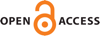Download this articleFor screen For printingRecent IssuesThe Journal About the Journal Editorial Board Editorial Interests Editorial Procedure Subscriptions Submission Guidelines Submission Page Policies for Authors Ethics Statement ISSN (electronic): 1364-0380 ISSN (print): 1465-3060 Author Index To Appear Other MSP JournalsHigh-energy harmonic maps and degeneration of minimal surfaces

### Charles Ouyang

Geometry & Topology 27 (2023) 1691–1746##### Abstract

Let $S$ be a closed surface of genus $g\ge 2$ and $\rho$ a maximal $\mathrm{PSL}\left(2,ℝ\right)×\mathrm{PSL}\left(2,ℝ\right)$ surface group representation. By a result of Schoen, there is a unique $\rho$–equivariant minimal surface $\stackrel{~}{\mathrm{\Sigma }}$ in ${ℍ}^{2}×{ℍ}^{2}$. We study the induced metrics on these minimal surfaces and prove the limits are precisely mixed structures. We prove a similar result for maximal surfaces in ${\mathrm{AdS}}^{3}$. In the second half of the paper, we provide a geometric interpretation: the minimal surfaces $\stackrel{~}{\mathrm{\Sigma }}$ degenerate to the core of a product of two $ℝ$–trees. As a consequence, we obtain a compactification of the space of maximal representations of ${\pi }_{1}\left(S\right)$ into $\mathrm{PSL}\left(2,ℝ\right)×\mathrm{PSL}\left(2,ℝ\right)$.

##### Keywords
minimal lagrangian, harmonic maps, mixed structures, higher Teichmüller space
##### Mathematical Subject Classification 2010
Primary: 49Q05, 53C43
##### Publication